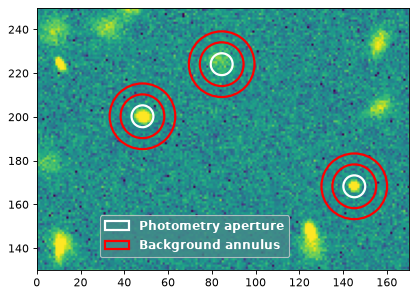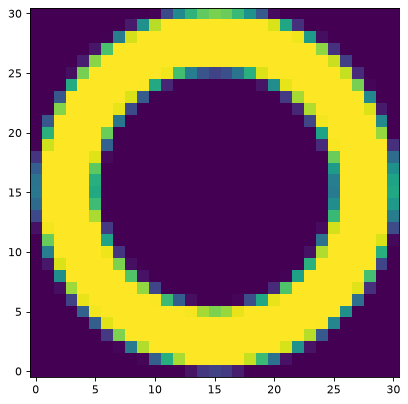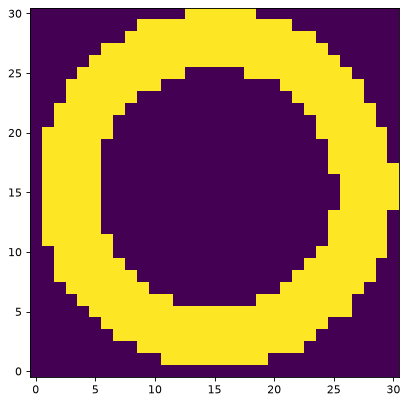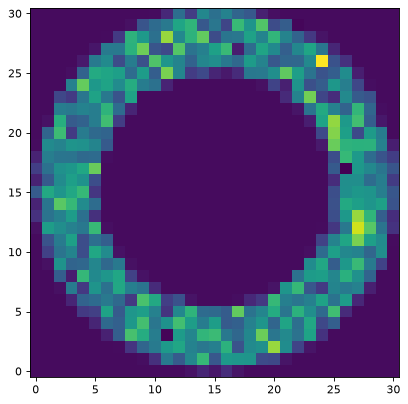Aperture Photometry (photutils.aperture)¶

Introduction¶

In Photutils, the aperture_photometry() function is the main tool to perform aperture photometry on an astronomical image for a given set of apertures.

Photutils provides several apertures defined in pixel or sky coordinates. The aperture classes that are defined in pixel coordinates are:

Each of these classes has a corresponding variant defined in sky coordinates:

To perform aperture photometry with sky-based apertures, one will need to specify a WCS transformation.

Users can also create their own custom apertures (see Defining Your Own Custom Apertures).

Creating Aperture Objects¶

The first step in performing aperture photometry is to create an aperture object. An aperture object is defined by a position (or a list of positions) and parameters that define its size and possibly, orientation (e.g., an elliptical aperture).

We start with an example of creating a circular aperture in pixel coordinates using the CircularAperture class:

>>> from photutils.aperture import CircularAperture
>>> positions = [(30., 30.), (40., 40.)]
>>> aperture = CircularAperture(positions, r=3.)

The positions should be either a single tuple of (x, y), a list of (x, y) tuples, or an array with shape Nx2, where N is the number of positions. The above example defines two circular apertures located at pixel coordinates (30, 30) and (40, 40) with a radius of 3 pixels.

Creating an aperture object in sky coordinates is similar. One first uses the SkyCoord class to define sky coordinates and then the SkyCircularAperture class to define the aperture object:

>>> from astropy import units as u
>>> from astropy.coordinates import SkyCoord
>>> from photutils.aperture import SkyCircularAperture
>>> positions = SkyCoord(l=[1.2, 2.3] * u.deg, b=[0.1, 0.2] * u.deg,
...                      frame='galactic')
>>> aperture = SkyCircularAperture(positions, r=4. * u.arcsec)

Note

Sky apertures are not defined completely in sky coordinates. They simply use sky coordinates to define the central position, and the remaining parameters are converted to pixels using the pixel scale of the image at the central position. Projection distortions are not taken into account. If the apertures were defined completely in sky coordinates, their shapes would not be preserved when converting to pixel coordinates.

Converting Between Pixel and Sky Apertures¶

The pixel apertures can be converted to sky apertures, and vice versa. To accomplish this, use the to_sky() method for pixel apertures, e.g.,:

>>> aperture = CircularAperture((10, 20), r=4.)
>>> sky_aperture = aperture.to_sky(wcs)

and the to_pixel() method for sky apertures, e.g.,:

>>> position = SkyCoord(1.2, 0.1, unit='deg', frame='icrs')
>>> aperture = SkyCircularAperture(position, r=4. * u.arcsec)
>>> pix_aperture = aperture.to_pixel(wcs)

Performing Aperture Photometry¶

After the aperture object is created, we can then perform the photometry using the aperture_photometry() function. We start by defining the aperture (at two positions) as described above:

>>> positions = [(30., 30.), (40., 40.)]
>>> aperture = CircularAperture(positions, r=3.)

We then call the aperture_photometry() function with the data and the apertures:

>>> import numpy as np
>>> from photutils.aperture import aperture_photometry
>>> data = np.ones((100, 100))
>>> phot_table = aperture_photometry(data, aperture)
>>> phot_table['aperture_sum'].info.format = '%.8g'  # for consistent table output
>>> print(phot_table)
id xcenter ycenter aperture_sum
pix     pix
--- ------- ------- ------------
1    30.0    30.0    28.274334
2    40.0    40.0    28.274334

This function returns the results of the photometry in an Astropy QTable. In this example, the table has four columns, named 'id', 'xcenter', 'ycenter', and 'aperture_sum'.

Since all the data values are 1.0, the aperture sums are equal to the area of a circle with a radius of 3:

>>> print(np.pi * 3. ** 2)
28.2743338823

Aperture and Pixel Overlap¶

The overlap of the aperture with the data pixels can be handled in different ways. For the default method (method='exact'), the exact intersection of the aperture with each pixel is calculated. The other options, 'center' and 'subpixel', are faster, but with the expense of less precision. For 'center', a pixel is considered to be entirely in or out of the aperture depending on whether its center is in or out of the aperture. For 'subpixel', pixels are divided into a number of subpixels, which are in or out of the aperture based on their centers. For this method, the number of subpixels needs to be set with the subpixels keyword.

This example uses the 'subpixel' method where pixels are resampled by a factor of 5 (subpixels=5) in each dimension:

>>> phot_table = aperture_photometry(data, aperture, method='subpixel',
...                                  subpixels=5)
>>> print(phot_table)
id xcenter ycenter aperture_sum
pix     pix
--- ------- ------- ------------
1    30.0    30.0        27.96
2    40.0    40.0        27.96

Note that the results differ from the true value of 28.274333 (see above).

For the 'subpixel' method, the default value is subpixels=5, meaning that each pixel is equally divided into 25 smaller pixels (this is the method and subsampling factor used in SourceExtractor).

The precision can be increased by increasing subpixels, but note that computation time will be increased.

Multiple Apertures at Each Position¶

While the Aperture objects support multiple positions, they must have a fixed size and shape (e.g., radius and orientation).

To perform photometry in multiple apertures at each position, one may input a list of aperture objects to the aperture_photometry() function. In this case, the apertures must all have identical position(s).

Suppose that we wish to use three circular apertures, with radii of 3, 4, and 5 pixels, on each source:

>>> radii = [3., 4., 5.]
>>> apertures = [CircularAperture(positions, r=r) for r in radii]
>>> phot_table = aperture_photometry(data, apertures)
>>> for col in phot_table.colnames:
...     phot_table[col].info.format = '%.8g'  # for consistent table output
>>> print(phot_table)
id xcenter ycenter aperture_sum_0 aperture_sum_1 aperture_sum_2
pix     pix
--- ------- ------- -------------- -------------- --------------
1      30      30      28.274334      50.265482      78.539816
2      40      40      28.274334      50.265482      78.539816

For multiple apertures, the output table column names are appended with the positions index.

Other apertures have multiple parameters specifying the aperture size and orientation. For example, for elliptical apertures, one must specify a, b, and theta:

>>> from photutils.aperture import EllipticalAperture
>>> a = 5.
>>> b = 3.
>>> theta = np.pi / 4.
>>> apertures = EllipticalAperture(positions, a, b, theta)
>>> phot_table = aperture_photometry(data, apertures)
>>> for col in phot_table.colnames:
...     phot_table[col].info.format = '%.8g'  # for consistent table output
>>> print(phot_table)
id xcenter ycenter aperture_sum
pix     pix
--- ------- ------- ------------
1      30      30     47.12389
2      40      40     47.12389

Again, for multiple apertures one should input a list of aperture objects, each with identical positions:

>>> a = [5., 6., 7.]
>>> b = [3., 4., 5.]
>>> theta = np.pi / 4.
>>> apertures = [EllipticalAperture(positions, a=ai, b=bi, theta=theta)
...              for (ai, bi) in zip(a, b)]
>>> phot_table = aperture_photometry(data, apertures)
>>> for col in phot_table.colnames:
...     phot_table[col].info.format = '%.8g'  # for consistent table output
>>> print(phot_table)
id xcenter ycenter aperture_sum_0 aperture_sum_1 aperture_sum_2
pix     pix
--- ------- ------- -------------- -------------- --------------
1      30      30       47.12389      75.398224      109.95574
2      40      40       47.12389      75.398224      109.95574

Background Subtraction¶

Global Background Subtraction¶

aperture_photometry() assumes that the data have been background-subtracted. If bkg is a float value or an array representing the background of the data (determined by Background2D or an external function), simply subtract the background:

>>> phot_table = aperture_photometry(data - bkg, aperture)

Local Background Subtraction¶

One often wants to estimate the local background around each source using a nearby aperture or annulus aperture surrounding each source. The simplest method for doing so would be to perform photometry in an annulus aperture to define the mean background level. Alternatively, one can use aperture masks to directly access the pixel values in an aperture (e.g., an annulus), and thus apply more advanced statistics (e.g., a sigma-clipped median within the annulus). We show examples of both below.

Simple mean within a circular annulus¶

For this example we perform the photometry in a circular aperture with a radius of 3 pixels. The local background level around each source is estimated as the mean value within a circular annulus of inner radius 6 pixels and outer radius 8 pixels. We start by defining the apertures:

>>> from photutils.aperture import CircularAnnulus
>>> aperture = CircularAperture(positions, r=3)
>>> annulus_aperture = CircularAnnulus(positions, r_in=6., r_out=8.)

We then perform the photometry in both apertures:

>>> apers = [aperture, annulus_aperture]
>>> phot_table = aperture_photometry(data, apers)
>>> for col in phot_table.colnames:
...     phot_table[col].info.format = '%.8g'  # for consistent table output
>>> print(phot_table)
id xcenter ycenter aperture_sum_0 aperture_sum_1
pix     pix
--- ------- ------- -------------- --------------
1      30      30      28.274334      87.964594
2      40      40      28.274334      87.964594

The aperture_sum_0 column refers to the first aperture in the list of input apertures (i.e., the circular aperture) and the aperture_sum_1 column refers to the second aperture (i.e., the circular annulus). Note that we cannot simply subtract the aperture sums because the apertures have different areas.

To calculate the mean local background within the circular annulus aperture, we need to divide its sum by its area. The mean value can be calculated by using the area() attribute:

>>> bkg_mean = phot_table['aperture_sum_1'] / annulus_aperture.area

The total background within the circular aperture is then the mean local background times the circular aperture area:

>>> bkg_sum = bkg_mean * aperture.area
>>> final_sum = phot_table['aperture_sum_0'] - bkg_sum
>>> phot_table['residual_aperture_sum'] = final_sum
>>> phot_table['residual_aperture_sum'].info.format = '%.8g'  # for consistent table output
>>> print(phot_table['residual_aperture_sum'])
residual_aperture_sum
---------------------
-7.1054274e-15
-7.1054274e-15

The result here should be zero because all the data values are 1.0 (the tiny difference from 0.0 is due to numerical precision).

Sigma-clipped median within a circular annulus¶

For this example we perform the photometry in a circular aperture with a radius of 5 pixels. The local background level around each source is estimated as the sigma-clipped median value within a circular annulus of inner radius 10 pixels and outer radius 15 pixels. We start by defining an example image and an aperture for three sources:

>>> from photutils.datasets import make_100gaussians_image
>>> from photutils.aperture import CircularAperture, CircularAnnulus
>>> data = make_100gaussians_image()
>>> positions = [(145.1, 168.3), (84.5, 224.1), (48.3, 200.3)]
>>> aperture = CircularAperture(positions, r=5)
>>> annulus_aperture = CircularAnnulus(positions, r_in=10, r_out=15)

Let’s plot the circular apertures (white) and circular annulus apertures (red) on the image:We can use aperture masks to directly access the pixel values in any aperture. Let’s do that for the annulus aperture:

The result is a list of ApertureMask objects, one for each aperture position. The values in these aperture masks are either 0 or 1 because we specified method='center'. Alternatively, one could use the “exact” (method='exact') mask, but it produces partial-pixel masks (i.e., values between 0 and 1) and thus one would need to use statistical functions that can handle partial-pixel weights. That introduces unnecessary complexity when the aperture is simply being used to estimate the local background. Whole pixels are fine, assuming you have a sufficient number of them on which to apply your statistical estimator.

Let’s focus on just the first annulus. Let’s plot its aperture mask:

>>> import matplotlib.pyplot as plt
>>> plt.colorbar()We can now use the photutils.aperture.ApertureMask.multiply() method to get the values of the aperture mask multiplied to the data. Since the mask values are 0 or 1, the result is simply the data values within the annulus aperture:

Let’s plot the annulus data:From this 2D array, you can extract a 1D array of data values (e.g., if you don’t care about their spatial positions, which is probably the most common case):

>>> annulus_data_1d = annulus_data[mask > 0]
>>> annulus_data_1d.shape
(394,)

You can then use your favorite statistical estimator on this 1D array to estimate the background level. Let’s calculate the sigma-clipped median:

>>> from astropy.stats import sigma_clipped_stats
>>> _, median_sigclip, _ = sigma_clipped_stats(annulus_data_1d)
>>> print(median_sigclip)
4.848212997882959

The total background within the circular aperture is then the local background level times the circular aperture area:

>>> background = median_sigclip * aperture.area
>>> print(background)
380.7777584296913

Above was a very pedagogical description of the underlying methods for local background subtraction for a single source. However, it’s quite straightforward to do this for all the sources in just a few lines of code. For this example, we’ll again use the sigma-clipped median of the pixels in the background annuli for the background estimates of each source:

>>> from astropy.stats import sigma_clipped_stats
>>> from photutils.aperture import aperture_photometry
>>> from photutils.aperture import CircularAperture, CircularAnnulus
>>> from photutils.datasets import make_100gaussians_image
>>>
>>> data = make_100gaussians_image()
>>> positions = [(145.1, 168.3), (84.5, 224.1), (48.3, 200.3)]
>>> aperture = CircularAperture(positions, r=5)
>>> annulus_aperture = CircularAnnulus(positions, r_in=10, r_out=15)
>>>
>>> bkg_median = []
...     annulus_data_1d = annulus_data[mask.data > 0]
...     _, median_sigclip, _ = sigma_clipped_stats(annulus_data_1d)
...     bkg_median.append(median_sigclip)
>>> bkg_median = np.array(bkg_median)
>>> phot = aperture_photometry(data, aperture)
>>> phot['annulus_median'] = bkg_median
>>> phot['aper_bkg'] = bkg_median * aperture.area
>>> phot['aper_sum_bkgsub'] = phot['aperture_sum'] - phot['aper_bkg']
>>> for col in phot.colnames:
...     phot[col].info.format = '%.8g'  # for consistent table output
>>> print(phot)
id xcenter ycenter aperture_sum annulus_median  aper_bkg aper_sum_bkgsub
pix     pix
--- ------- ------- ------------ -------------- --------- ---------------
1   145.1   168.3    1131.5794       4.848213 380.77776       750.80166
2    84.5   224.1    746.16064      5.0884354 399.64478       346.51586
3    48.3   200.3    1250.2186      4.8060599 377.46706        872.7515

Error Estimation¶

If and only if the error keyword is input to aperture_photometry(), the returned table will include a 'aperture_sum_err' column in addition to 'aperture_sum'. 'aperture_sum_err' provides the propagated uncertainty associated with 'aperture_sum'.

For example, suppose we have previously calculated the error on each pixel’s value and saved it in the array error:

>>> positions = [(30., 30.), (40., 40.)]
>>> aperture = CircularAperture(positions, r=3.)
>>> data = np.ones((100, 100))
>>> error = 0.1 * data

>>> phot_table = aperture_photometry(data, aperture, error=error)
>>> for col in phot_table.colnames:
...     phot_table[col].info.format = '%.8g'  # for consistent table output
>>> print(phot_table)
id xcenter ycenter aperture_sum aperture_sum_err
pix     pix
--- ------- ------- ------------ ----------------
1      30      30    28.274334       0.53173616
2      40      40    28.274334       0.53173616

'aperture_sum_err' values are given by:

$\Delta F = \sqrt{\sum_{i \in A} \sigma_{\mathrm{tot}, i}^2}$

where $$A$$ are the non-masked pixels in the aperture, and $$\sigma_{\mathrm{tot}, i}$$ is the input error array.

In the example above, it is assumed that the error keyword specifies the total error – either it includes Poisson noise due to individual sources or such noise is irrelevant. However, it is often the case that one has calculated a smooth “background-only error” array, which by design doesn’t include increased noise on bright pixels. To include Poisson noise from the sources, we can use the calc_total_error() function.

Let’s assume we have a background-only image called bkg_error. If our data are in units of electrons/s, we would use the exposure time as the effective gain:

>>> from photutils.utils import calc_total_error
>>> effective_gain = 500  # seconds
>>> error = calc_total_error(data, bkg_error, effective_gain)
>>> phot_table = aperture_photometry(data - bkg, aperture, error=error)

Pixels can be ignored/excluded (e.g., bad pixels) from the aperture photometry by providing an image mask via the mask keyword:

>>> data = np.ones((5, 5))
>>> aperture = CircularAperture((2, 2), 2.)
>>> data[2, 2] = 100.  # bad pixel
>>> t1['aperture_sum'].info.format = '%.8g'  # for consistent table output
>>> print(t1['aperture_sum'])
aperture_sum
------------
11.566371

The result is very different if a mask image is not provided:

>>> t2 = aperture_photometry(data, aperture)
>>> t2['aperture_sum'].info.format = '%.8g'  # for consistent table output
>>> print(t2['aperture_sum'])
aperture_sum
------------
111.56637

Aperture Photometry Using Sky Coordinates¶

As mentioned in Creating Aperture Objects, performing photometry using apertures defined in sky coordinates simply requires defining a “sky” aperture at positions defined by a SkyCoord object. Here we show an example of photometry on real data using a SkyCircularAperture.

We start by loading a Spitzer 4.5 micron image of a region of the Galactic plane:

>>> import astropy.units as u
>>> from astropy.wcs import WCS

The catalog contains (among other things) the Galactic coordinates of the sources in the image as well as the PSF-fitted fluxes from the official Spitzer data reduction. We define the apertures positions based on the existing catalog positions:

>>> positions = SkyCoord(catalog['l'], catalog['b'], frame='galactic')
>>> aperture = SkyCircularAperture(positions, r=4.8 * u.arcsec)

Now perform the photometry in these apertures on the data. The wcs object contains the WCS transformation of the image obtained from the FITS header. It includes the coordinate frame of the image and the projection from sky to pixel coordinates. The aperture_photometry function uses the WCS information to automatically convert the apertures defined in sky coordinates into pixel coordinates:

>>> phot_table = aperture_photometry(data, aperture, wcs=wcs)

The Spitzer catalog also contains the official fluxes for the sources, so we can compare to our fluxes. Because the Spitzer catalog fluxes are in units of mJy and the data are in units of MJy/sr, we need to convert units before comparing the results. The image data have a pixel scale of 1.2 arcsec/pixel.

>>> import astropy.units as u
>>> factor = (1.2 * u.arcsec) ** 2 / u.pixel
>>> fluxes_catalog = catalog['f4_5']
>>> converted_aperture_sum = (phot_table['aperture_sum'] *
...                           factor).to(u.mJy / u.pixel)

Finally, we can plot the comparison of the photometry:

>>> import matplotlib.pyplot as plt
>>> plt.scatter(fluxes_catalog, converted_aperture_sum.value)
>>> plt.xlabel('Spitzer catalog PSF-fit fluxes ')
>>> plt.ylabel('Aperture photometry fluxes')Despite using different methods, the two catalogs are in good agreement. The aperture photometry fluxes are based on a circular aperture with a radius of 4.8 arcsec. The Spitzer catalog fluxes were computed using PSF photometry. Therefore, differences are expected between the two measurements.

All PixelAperture objects have a to_mask() method that returns a list of ApertureMask objects, one for each aperture position. The ApertureMask object contains a cutout of the aperture mask and a BoundingBox object that provides the bounding box where the mask is to be applied. It also provides a to_image() method to obtain an image of the mask in a 2D array of the given shape, a cutout() method to create a cutout from the input data over the mask bounding box, and an multiply() method to multiply the aperture mask with the input data to create a mask-weighted data cutout. All of these methods properly handle the cases of partial or no overlap of the aperture mask with the data.

Let’s start by creating an aperture object:

>>> from photutils.aperture import CircularAperture
>>> positions = [(30., 30.), (40., 40.)]
>>> aperture = CircularAperture(positions, r=3.)

Now let’s create a list of ApertureMask objects using the to_mask() method:

We can now create an image with of the first aperture mask at its position:

We can also create a cutout from a data image over the mask domain:

We can also create a mask-weighted cutout from the data. Here the circular aperture mask has been multiplied with the data:

The aperture_photometry() function can perform aperture photometry in arbitrary apertures. This function accepts any Aperture-derived objects, such as CircularAperture. This makes it simple to extend functionality: a new type of aperture photometry simply requires the definition of a new Aperture subclass.

All PixelAperture subclasses must define a bounding_boxes property and to_mask() and plot() methods. They may also optionally define an area property. All SkyAperture subclasses must only implement a to_pixel() method.

• bounding_boxes: The minimal bounding box for the aperture. If the aperture is scalar, then a single BoundingBox is returned. Otherwise, a list of BoundingBox is returned.

• area: An optional property defining the exact analytical area (in pixels**2) of the aperture.

• to_mask(): Return a mask for the aperture. If the aperture is scalar, then a single ApertureMask is returned. Otherwise, a list of ApertureMask is returned.

• plot(): A method to plot the aperture on a matplotlib.axes.Axes instance.

Reference/API¶

This subpackage contains tools to perform aperture photometry.

Functions¶

 aperture_photometry(data, apertures[, …]) Perform aperture photometry on the input data by summing the flux within the given aperture(s).

Classes¶

 Aperture() Abstract base class for all apertures. ApertureMask(data, bbox) Class for an aperture mask. BoundingBox(ixmin, ixmax, iymin, iymax) A rectangular bounding box in integer (not float) pixel indices. CircularAnnulus(positions, r_in, r_out) A circular annulus aperture defined in pixel coordinates. CircularAperture(positions, r) A circular aperture defined in pixel coordinates. Mixin class to create masks for circular and circular-annulus aperture objects. EllipticalAnnulus(positions, a_in, a_out, b_out) An elliptical annulus aperture defined in pixel coordinates. EllipticalAperture(positions, a, b[, theta]) An elliptical aperture defined in pixel coordinates. Mixin class to create masks for elliptical and elliptical-annulus aperture objects. Abstract base class for apertures defined in pixel coordinates. RectangularAnnulus(positions, w_in, w_out, h_out) A rectangular annulus aperture defined in pixel coordinates. RectangularAperture(positions, w, h[, theta]) A rectangular aperture defined in pixel coordinates. Mixin class to create masks for rectangular or rectangular-annulus aperture objects. Abstract base class for all apertures defined in celestial coordinates. SkyCircularAnnulus(positions, r_in, r_out) A circular annulus aperture defined in sky coordinates. SkyCircularAperture(positions, r) A circular aperture defined in sky coordinates. SkyEllipticalAnnulus(positions, a_in, a_out, …) An elliptical annulus aperture defined in sky coordinates. SkyEllipticalAperture(positions, a, b[, theta]) An elliptical aperture defined in sky coordinates. SkyRectangularAnnulus(positions, w_in, …) A rectangular annulus aperture defined in sky coordinates. SkyRectangularAperture(positions, w, h[, theta]) A rectangular aperture defined in sky coordinates.